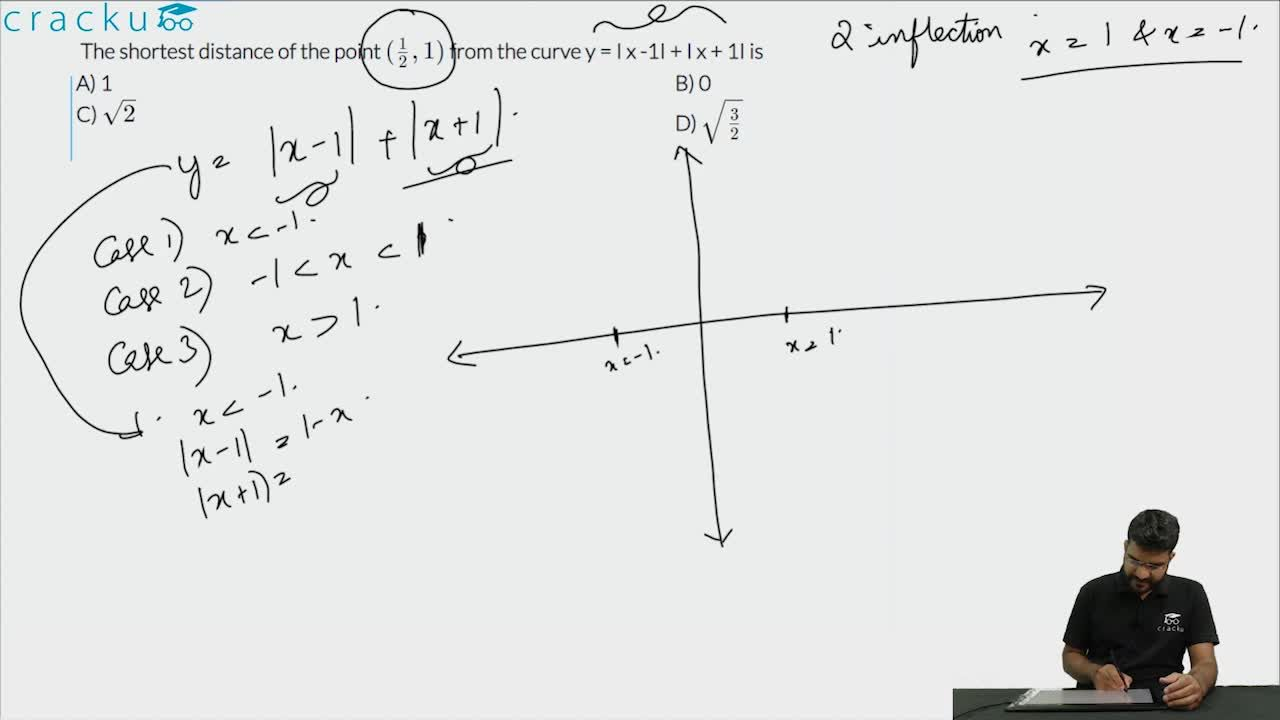Question 96

# The shortest distance of the point $$(\frac{1}{2},1)$$ from the curve y = I x -1I + I x + 1I is

Solution

The graph of the given function is as shown below.

We can see that the shortest distance of the point (1/2, 1) will be 1 unit.

### View Video Solution